# Chemical Bonding

## 2008

#### Question 1

State which is not a common characteristic of an electrovalent compound?

1. High melting point.
2. Conducts electricity when molten.
3. Consists of oppositely charged ions.
4. Ionizes when dissolved in water.

State why CCl4 is a non-electrolyte.

Ionizes when dissolved in water.
Reason — Electrovalent compounds undergoes electrolytic dissociation on passage of electric current. The process involves separation of ions already present in the ionic compound.

As CCl4 is a non-polar covalent compound so it has molecules and not ions. Hence, it does not undergo electrolytic dissociation and is a non-electrolyte.

#### Question 2

State the terms defined in each case:

A bond formed by – (a) a shared pair of electrons, each bonding atom contributing one electron to the pair.

(b) a shared pair of electrons with both electrons coming from the same atom.

(a) Covalent bond

(b) Coordinate bond

## 2009

#### Question 1

The one which is composed of all the three kinds of bond [ionic, covalent and coordinate bond] is:

1. Sodium chloride
2. Ammonia
3. Carbon tetrachloride
4. Ammonium chloride

Ammonium chloride
Reason — When ammonium chloride NH4Cl is formed cation NH4+ (having 3 covalent and one coordinate bond) and anion Cl- are attracted towards each other, due to electrical charge existing between them ionic bond is formed. Thus, ammonium chloride is a good example of compound having all three types of bonds i.e., covalent, coordinate and ionic bond.

#### Question 2

Draw the structural formula of carbon tetrachloride and state the type of bond present in it.

Structural formula of carbon tetrachloride is given below: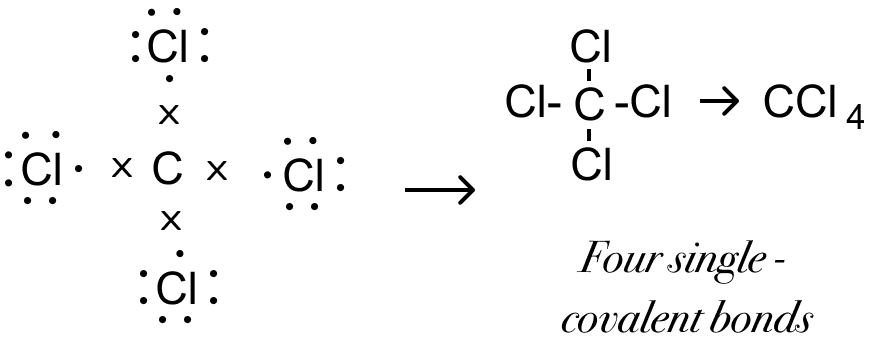Covalent bond is present in carbon tetrachloride.

## 2010

#### Question 1

Select the correct answer from A, B, C and D — Metals lose electrons during ionization — this change is called:

1. Oxidation
2. Reduction
3. Redox
4. Displacement

Oxidation
Reason — Oxidation is the process when an atom or ion loses electrons in order to attain a stable state.

#### Question 2

Select the right answer from the choices — covalent bond / ionic bond / covalent & coordinate bond for each of the following —

(i) Sodium chloride

(ii) Ammonium ion

(iii) Carbon tetrachloride

(i) Sodium chloride — Ionic bond

(ii) Ammonium ion — Covalent and coordinate bond

(iii) Carbon tetrachloride — Covalent bond

## 2011

#### Question 1

(i) In covalent compounds, the bond is formed due to ............... [sharing/transfer] of electrons.

(ii) Electrovalent compounds have a ............... [low/high] boiling point.

(iii) A molecule of ............... contains a triple bond, [hydrogen, ammonia, nitrogen]

(i) In covalent compounds, the bond is formed due to sharing of electrons.

(ii) Electrovalent compounds have a high boiling point.

(iii) A molecule of nitrogen contains a triple bond.

#### Question 2

Draw an electron dot diagram: showing the lone pair effect for formation of — NH41+ ion from NH3 gas and H1+.

Electron dot diagram showing the lone pair effect for formation of NH41+ ion from NH3 gas and H1+ is given below: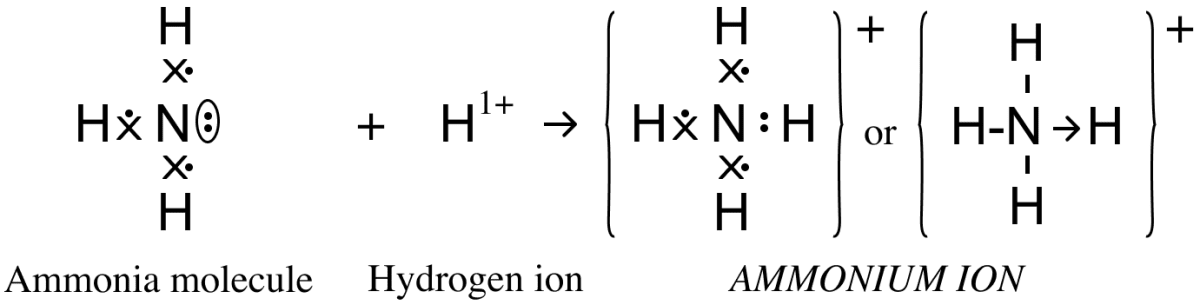#### Question 3

Give reasons — Hydrogen chloride can be termed as a polar covalent compound.

In hydrogen chloride, the strong nuclear charge of the chlorine atom attracts the shared electron pair towards itself, i.e., negative charge shifts towards the chlorine atom thereby developing a slight negative charge on it. The hydrogen atom develops a slight positive charge. Therefore, a polar covalent bond is formed as shown below :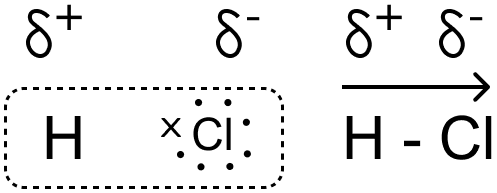## 2012

#### Question 1

Draw an electron dot diagram of the structure of — hydronium ion. State the type of bonding present in it.

Electron dot diagram showing the structure of Hydronium ion is given below: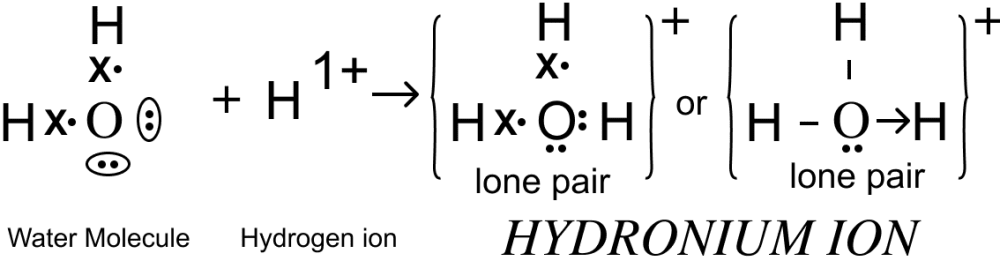Co-ordinate bond is present in hydronium ion.

#### Question 2

There are three elements E, F, G with atomic numbers 19, 8 and 17 respectively. Give the molecular formula of the compound formed between E and G and state the type of chemical bond in this compound.

EG is the chemical formula.
The electronic configuration of E is (2,8,8,1) and G is (2,8,7). We observe that E tends to donate one electron and G tends to gain one electron to attain a stable state. Hence, the the two oppositely charged ions attract each other to form an ionic compound EG.
Ionic bond will be formed.

## 2013

#### Question 1

A chemical term for: A bond formed by a shared pair of electrons with both electrons coming from the same atom.

Coordinate bond

#### Question 2

Among the compounds identify the compound that has all three bonds [ionic, covalent and coordinate bond].

1. Ammonia
2. Ammonium chloride
3. Sodium hydroxide
4. Calcium chloride

Ammonium chloride

#### Question 3

State which is not a typical property of an ionic compound?

1. High melting point.
2. Conducts electricity in molten and in the aqueous solution state.
3. Are insoluble in water.
4. Exist as oppositely charged ions even in the solid state.

Are insoluble in water
Reason — Ionic compounds are soluble in water. They are insoluble in organic solvents. Water [polar solvent] has a high dielectric constant i.e. capacity to weaken the force of attraction, thus resulting in free ions.
Organic solvents [non-polar] have low dielectric constants and do not cause dissolution.

#### Question 4

Compare carbon tetrachloride and sodium chloride with regard to solubility in water and electrical conductivity.

Carbon tetrachlorideSodium chloride
Solubility in waterInsoluble in waterSoluble in water
Electrical conductivityNon ConductorGood conductors in aqueous state or molten state

## 2014

#### Question 1

Compound 'X' consists of only molecules. 'X' will have —

1. A Crystalline hard structure
2. A low melting and boiling point
3. An ionic bond
4. A strong force of attraction between it's molecules.

A low melting and boiling point
Reason — There are weak Vander Waals forces of attraction between molecules. Thus, less amount of energy is required to break these forces of attraction.

#### Question 2

The molecule which contains a triple covalent bond is:

1. ammonia
2. methane
3. water
4. nitrogen

nitrogen
Reason — The valency of nitrogen element is 3. Nitrogen needs three electrons to attain stable octet structure of nearest noble gas - Ne [2,8].
Each of the two N atoms contributes three electron so as to have three shared pair of electrons between them. Both atoms attain stable – octet structure, resulting in the – formation of a – triple covalent bond [N≡N] between them

#### Question 3

Give word/phrase for : Formation of ions from molecules.

Ionisation

#### Question 4

Give a reason why covalent compounds exist as gases, liquids or soft solids?

Covalent compounds exist as gases, liquids or soft solids as they have weak force of attraction between their molecules.

## 2015

#### Question 1

Bonding in which —

1. CCl4
2. H2
3. HCl
4. NH4Cl

— involves coordinate bonding.

NH4Cl
Reason — The bond formed between the nitrogen atom in ammonia and the chloride ion is a coordi­nate bond.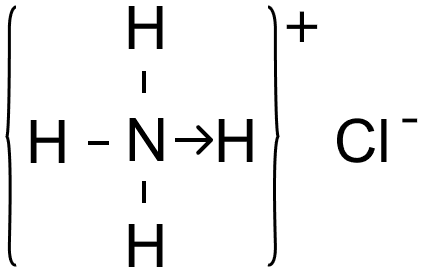#### Question 2

Give scientific reasons: Carbon tetrachloride does not conduct electricity.

Carbon tetrachloride is made up of molecules and not free ions hence, it does not conduct electricity.

#### Question 3

Explain the bonding in methane molecule using electron dot structure.

Formation of methane molecule — The valency of C is 4 and that of H is 1. Carbon needs four electrons to attain stable octet and hydrogen needs one electron to attain it's stable duplet state. Hence, one carbon atom shares four electron pairs - one with each of the four atoms of hydrogen. Hence, forms four single covalent bonds.

Electron Dot Structure of methane molecule: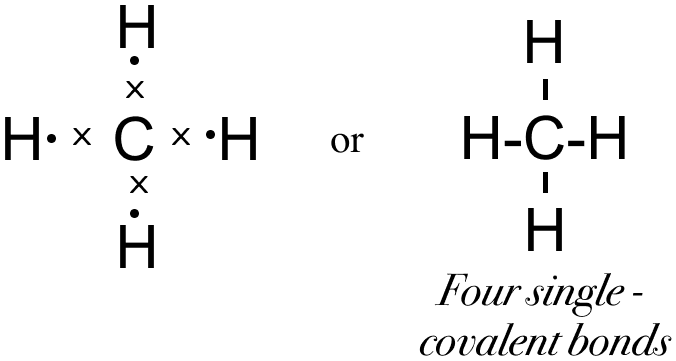#### Question 4

An element L consists of molecules.

(i) What type of bonding is present in the particles that make up L?

(ii) When L is heated with iron metal, it forms a compound FeL. What chemical term would you use to describe the change undergone by L?

(i) Covalent bonding is present in L as the bond is formed between same atoms.

(ii) On heating, Fe undergoes oxidation and loses two electron as follows :

Fe - 2e– ⟶ Fe2+

Two electrons are taken up by L and it undergoes reduction

## 2016

#### Question 1

Fill in the blanks from the choices given :

Electrovalent compounds have .............. [high/low] melting points.

Electrovalent compounds have high melting points.

#### Question 2

Elements — W, X, Y & Z have electronic configurations:
W = 2,8,1
X = 2,8,7
Y = 2,5
Z = 1

(i) What type of bond is formed between:
(a) W and X
(b) Y and Z

(ii) What is the formula of the compound formed between :
(a) X and Z
(b) W and X

(i) Type of bond formed

(a) W (2,8,1) and X (2,8,7) — Electrovalent bond is formed as W donates 1 electron and X takes up that electron and both attain a stable octet.

(b) Y (2, 5) and Z (1) — Covalent bond as electron sharing takes place between the two elements. The valency of Z element is 1 and that of Y is 3. Z needs one electrons to attain stable duplet structure of nearest noble gas - He  and Y needs three electrons to attain stable octet structure of nearest noble gas - Ne [2,8]
One Y atom shares three electron pairs one with each of the three atoms of Z such that Z acquires a duplet configuration and Y attain a stable octet configuration resulting in the formation of three single covalent bonds.

(ii) Formula of the compound formed

(a) X (2,8,7) and Z (1) — XZ as X needs one 1 electron to complete it's octet and Z needs needs one electron to complete it's duplet hence, they both share one electron pair and forms ZX compound.

(b) W (2,8,1) and X (2,8,7) — WX as W has the tendency to donate one electron and gain stability whereas X has the tendency to gain one electron to be stable. Hence, W donates one electron and X takes it and form WX compound.

#### Question 3

Draw an electron dot diagram to show the formation of ammonium ion [N = 7, H = 1]

Electron dot diagram showing the formation of ammonium ion is given below:## 2017

#### Question 1

Fill in the blanks:

The compound that does not have a lone pair of electrons is ............... [water, ammonia, carbon tetrachloride].

The compound that does not have a lone pair of electrons is carbon tetrachloride.

#### Question 2

State which of the following is a common characteristic of a covalent compound.

1. High melting point
2. Consists of molecules
3. Always soluble in water
4. Conducts electricity when it is in the molten state.

Consists of molecules
Reason — In covalent compound, valence shell electrons are mutually shared by atom of each element to achieve a stable electronic configuration. As there is no transfer of electrons between the atoms so the atoms remain electrically neutral. Hence they don't form ions and covalent compounds consists of molecules.

#### Question 3

State the type of bonding in the following molecules:

(i) Water

(ii) Calcium Oxide

(i) Covalent bonding
Reason — The valency of hydrogen element is 1 and that of oxygen is 2. Hydrogen needs one electrons to attain stable duplet structure of nearest noble gas - He  and oxygen needs two electrons to attain stable octet structure of nearest noble gas - Ne [2,8]
Each of the two hydrogen atoms shares an electron pair with the oxygen atom such that hydrogen acquires a duplet configuration and oxygen an octet configuration resulting in the formation of two single covalent bonds [H-O-H] in the molecule of water.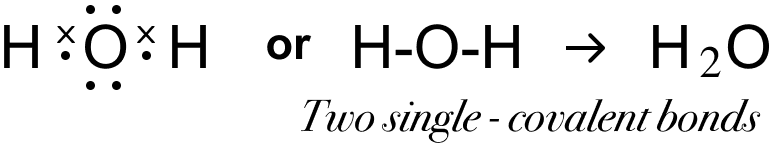(ii) Electrovalent bonding
Reason — The valency of calcium (2,8,8,2) element and that of oxygen (2,6) is 2. Calcium donates two electrons and oxygen takes up those two electrons and electrovalent bond is formed.

#### Question 4

Draw an electron dot diagram to show the formation of each of the following compounds —

(i) Methane

(ii) Magnesium chloride

[H = 1, C = 6, Mg = 12, Cl = 17]

(i) Electron dot diagram showing the formation of Methane is given below:(ii) Electron dot diagram showing the formation of Magnesium chloride is given below: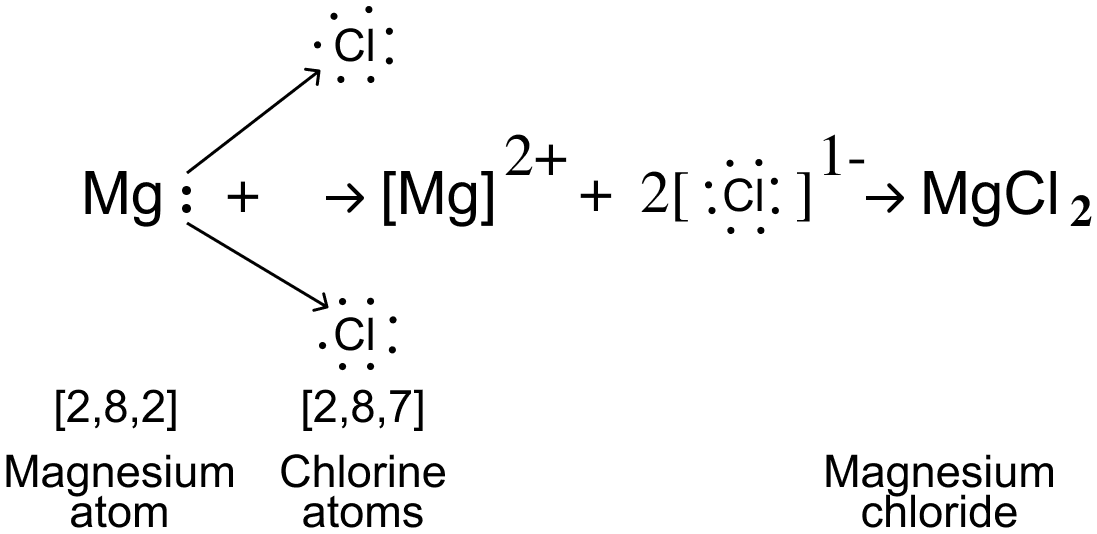## 2018

#### Question 1

Give one word or a phrase for — Process of formation of ions from molecules which are not in the ionic state.

Ionization

#### Question 2

Give a reason for — Ionic compounds have a high melting point.

Ionic compounds have ionic bonds so there exists a strong force of attraction between the oppositely charged ions, so a large amount of energy is required to break the strong bonding between the ions. Hence, ionic compounds have a high melting point.

#### Question 3

Fill in the blank with the correct choice given in the bracket —

Ionic or electrovalent compounds do not conduct electricity in their ............... state. [fused/solid]

Ionic or electrovalent compounds do not conduct electricity in their solid state.

#### Question 4

State the meaning of 'lone pair of electrons'. Draw the electron dot diagram of Hydronium ion. [H = 1, O = 8]

The pair of electrons not shared by any other atom are called lone pair of electrons. Electron dot diagram of Hydronium ion is shown below:## 2019

#### Question 1

Give the appropriate term for : The covalent bond in which the electrons are shared equally between the combining atoms.

Non-polar covalent bond

#### Question 2

Draw the electron dot structure of :

(i) Nitrogen molecule [N=7]

(ii) Sodium chloride [Na=11, Cl=17]

(iii) Ammonium ion [N=7, H=1]

(i) Electron dot structure of Nitrogen molecule is shown below: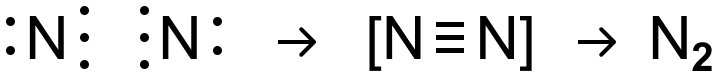(ii) Electron dot structure of Sodium chloride is shown below: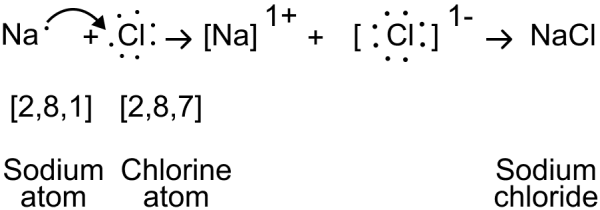(iii) Electron dot structure of Ammonium ion is shown below:## 2020

#### Question 1

Choose the correct answer from the options given :

A compound with low boiling point is :

1. Sodium chloride
2. Calcium chloride
3. Potassium chloride
4. Carbon tetrachloride

Carbon tetrachloride
Reason — Carbon Tetrachloride is a covalent compound and Covalent Compounds have low boiling point.

#### Question 2

Give one word or a phrase for : The chemical bond formed by a shared pair of electrons, each bonding atom contributing one electron to the pair.

Covalent bond

#### Question 3

Fill in the blanks from the choices given :

The polar covalent compound in gaseous state that does not conduct electricity is ............... [carbon tetrachloride, ammonia, methane].

The polar covalent compound in gaseous state that does not conduct electricity is ammonia.

#### Question 4

Draw the electron dot diagram for the compounds given. Represent the electrons by [.] and [x] in the diagram.

[Ca=20, O=8, Cl=17, H=1]

(i) Calcium oxide

(ii) Chlorine molecule

(iii) Water molecule

(i) Electron dot diagram of Calcium oxide is shown below: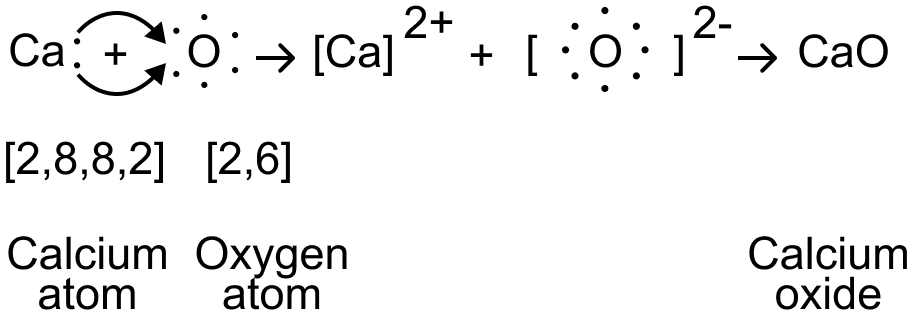(ii) Electron dot diagram of Chlorine molecule is shown below: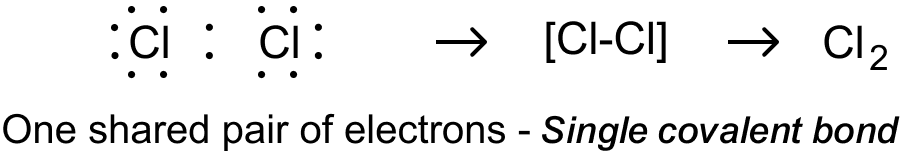(iii) Electron dot diagram of Water molecule is shown below:#### Question 1

State the force which holds two or more atoms together as a stable molecule.

Chemical bond is the force which holds two or more atoms together in a stable molecule.

#### Question 2

Draw the geometrical atomic structure representing the electronic configuration of atoms of elements of :

1. Period-2
1. group 14 [IVA] — carbon [at no. 6]
2. group 15 [VA] — nitrogen [at. no. 7]
3. group 16 [VI A] — oxygen [at no. 8]
2. Period-3
1. group 1[IA] — sodium [at no. 11]
2. group 2[IIA] — magnesium [at. no. 12]
3. group 17[VIIA]— chlorine [at. no. 17]
3. Period-4
1. group 2[IIA] — calcium [at. no. 20].

Period-2

(i) Group 14 [IV A] — Carbon[at no. 6]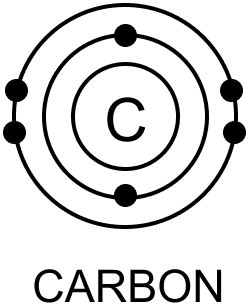(ii) Group 15 [VA] — Nitrogen [at. no. 7]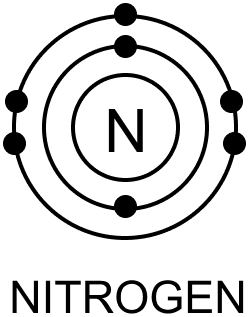(iii) Group 16 [VI A] — Oxygen [at no. 8]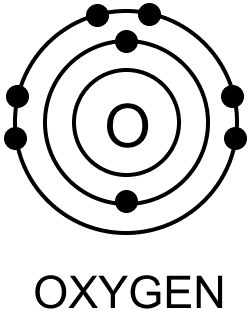Period-3

(i) Group 1[IA] — Sodium [at no. 11]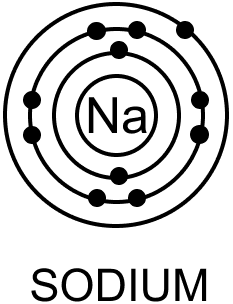(ii) Group 2[IIA] — Magnesium [at. no. 12]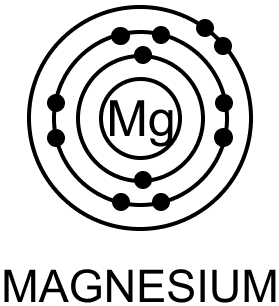(iii) Group 17[VIIA] — Chlorine [at. no. 17]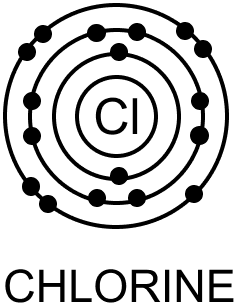Period-4

(i) group 2[IIA] — Calcium [at. no. 20].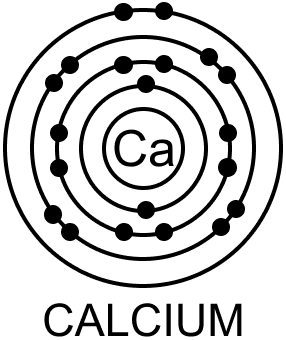#### Question 3

What is meant by the term 'chemical bond' and 'chemical bonding'.

Chemical bond — The linkage or force which acts between two or more atoms to hold them together as a stable molecule is called a chemical bond.

Chemical bonding — It is the formation of a chemical bond between two or more atoms to hold them together as a stable molecule resulting in a chemical compound.

#### Question 4

State why noble gases are unreactive while atoms of elements other than noble gases are chemically reactive.

Noble gases are unreactive because they have stable electronic configuration i.e., their outer most shell is complete and are stable hence they do not react. On the other hand elements other than noble gases have unstable electronic configuration i.e., their outermost shell is incomplete and they lose or gain electrons in order attain a stable state.

#### Question 5

State the reasons for chemical bonding between two atoms and the methods involved for achieving the same. State how 'duplet and octet' rules are involved for an atom to achieve stable electronic configuration.

The reasons for chemical bonding between two atoms is the tendency of elements to acquire the nearest noble gas configuration in their outermost orbit and become stable.

There are three methods in which atoms can achieve a stable configuration :

1. The transfer of one or more electrons from one atom to the other form an electrovalent (or ionic) bond.
2. Sharing of one, two or three pairs of electrons between two atoms to form a covalent (or molecular) bond.
3. When the shared pair of electron pairs are contributed by only one of the combining atoms, the bond formed is known as coordinate (or dative) bond.

For an atom to achieve stable electronic configuration it must have :

Either two electrons in it's outermost shell (nearest noble gas – He) — Duplet rule

Or

Eight electrons in it's outermost shell (all noble gases other than He) — Octet rule.

#### Question 6

State the type of compounds formed by transfer of valence electrons from one atom to another, and explain the method of formation of the same. State the role of 'cations' and 'anions' in their formation.

The type of compounds formed by transfer of valence electrons from one atom to another are Ionic or Electrovalent compounds.

Atoms of metallic elements that have 1, 2 or 3 valence electrons, can lose electron(s) to atoms of non-metallic elements, which have 7, 6 or 5 electrons respectively in their outermost shell and thereby forming an electrovalent compound.

After the transfer of electron(s) both the combining atoms acquire the electronic configuration of the nearest inert gas.

A metallic atom, which loses electron(s) becomes a positively charged ion and is known as a 'cation' and a non-metallic atom, which gains electrons(s) becomes a negatively charged ion and is known as an 'anion'.

The role of cation and anion is that they are oppositely charged and so they attract each other and form a chemical bond which is known as ionic or electrovalent bond.

#### Question 7

Define the terms:

(i) Electrovalent or ionic bond

(ii) Electrovalent or ionic compound.

Electrovalent or ionic bond — The chemical bond formed between two atoms by transfer of one or more electrons from the atom of a metallic (electropositive) element to an atom of a non-metallic (electronegative) element.

Electrovalent or ionic compound — The chemical compound formed as a result of transfer of one or more electrons from the atom of a metallic (electropositive) element to an atom of a non-metallic (electronegative) element.

#### Question 8

What is meant by the term 'electrovalency'. State why Na [at. no. 11] has a electropositive valency of +1 and Cl (at. no. 17) an electronegative valency of -1.

The number of electrons that an atom of an element loses or gains to form a electrovalent bond is called it's electrovalency.

The electronic configuration of Na (at. no. 11) = 2, 8, 1 and in order to attain the nearest noble gas electronic configuration i.e., Ne (2,8) it tends to lose 1 electron, hence, it is said that it has electropositive valency of +1.

The electronic configuration of Cl (at. no. 17) = 2, 8, 7 and in order to attain the nearest noble gas electronic configuration i.e., Ar (2, 8, 8) it tends to gain 1 electron, hence, it is said that it has electronegative valency of -1.

#### Question 9

State three differences between 'X' and 'X1+' i.e. an atom and an ion.

Differences between 'X' and 'X1+ are :

Atoms – 'X'Ions – 'X1+'
Electrically neutral particlesElectrically charged particles [cations, anions]
May or may not exist independentlyExist independently in solution
Outermost shell may or may not have duplet or octet.Outermost shell have complete duplet or octet.

#### Question 10

Explain the terms 'oxidation' and reduction' with reference to an atom or ion.

Oxidation — It is the process when an atom or ion loses one or more electrons i.e, X - 1e- ⟶ X1+

For example,

Na - 1e- ⟶ Na1+
Zn - 2e- ⟶ Zn2+
S2- - 2e- ⟶ S

Reduction — It is the process when an atom or ion gains one or more electrons i.e, Y + 1e- ⟶ Y1-

For example,

S + 2e- ⟶ S2-
Cu2+ + 2e- ⟶ Cu
Fe3+ + 1e- ⟶ Fe2+

#### Question 11

State which of the following are oxidation reactions and which are reduction reactions.

(a) (i) Cu ⟶ Cu2+ + 2e-

(ii) Cu2+ + 2e- ⟶ Cu

(iii) Sn4+ + 2e- ⟶ Sn2+

(iv) 2Cl- ⟶ Cl2 + 2e-

(v) Fe2+ ⟶ Fe3+ + 1e-

(vi) X + 2e- ⟶ X2-

(vii) Y - 1e- ⟶ Y1+

(viii) Z3+ + 1e- ⟶ Z2+

(b) (i) Zn ⟶ Zn2+

(ii) S ⟶ S2-

(iii) Sn2+ ⟶ Sn

(iv) Fe2+ ⟶ Fe3+

(a) (i) Cu ⟶ Cu2+ + 2e-Oxidation Reaction

(ii) Cu2+ + 2e- ⟶ Cu — Reduction Reaction

(iii) Sn4+ + 2e- ⟶ Sn2+Reduction Reaction

(iv) 2Cl- ⟶ Cl2 + 2e-Oxidation Reaction

(v) Fe2+ ⟶ Fe3+ + 1e-Oxidation Reaction

(vi) X + 2e- ⟶ X2-Reduction Reaction

(vii) Y - 1e- ⟶ Y1+Oxidation Reaction

(viii) Z3+ + 1e- ⟶ Z2+Reduction Reaction

(b) (i) Zn ⟶ Zn2+ Oxidation Reaction

(ii) S ⟶ S2-Reduction Reaction

(iii) Sn2+ ⟶ Sn — Reduction Reaction

(iv) Fe2+ ⟶ Fe3+Oxidation Reaction

#### Question 12

Explain with the help of :

(i) an ionic equation

(ii) electron dot structural diagram

the formation of the following :

(a) Sodium chloride

(b) Calcium oxide

(c) Magnesium chloride.

(at. nos. Na = 11, Cl = 17, Ca = 20, O = 8, Mg = 12)

(a) Sodium Chloride :

Ionic equation

Na - 1e- ⟶ Na1+ [oxidation]
[atom]      [cation]
[2,8,1]       [2,8]

Cl + 1e- ⟶ Cl1- [reduction]
[atom]      [anion]
[2,8,7]       [2,8,8]

Na + Cl ⟶ Na1+ Cl1- ⟶ NaCl

Electron dot structural diagram(b) Calcium Oxide

Ionic Equation

Ca - 2e- ⟶ Ca2+ [oxidation]
[atom]      [cation]
[2,8,8,2]     [2,8,8]

O + 2e- ⟶ O2- [reduction]
[atom]      [anion]
[2,6]          [2,8]

Ca + O ⟶ Ca2+O2- ⟶ CaO

Electron dot structural diagram(c) Magnesium chloride.

Ionic Equation

Mg - 2e- ⟶ Mg2+ [oxidation]
[atom]      [cation]
[2,8,2]       [2,8]

2Cl + 2e- ⟶ 2Cl- [reduction]
[atom]      [anion]
[2,8,7]       [2,8,8]

Mg + 2Cl ⟶ Mg2+2Cl- ⟶ MgCl2

Electron dot structural diagram#### Question 13.1

Fill in the blanks with appropriate word/s from the brackets :

NaCl an electrovalent compound is formed as a result of transfer of ............... (one, two, three) valence electrons from metallic sodium to non-metallic chlorine atom. CaO is similarly formed as a result of transfer of ............... (one, two, three) valence electron/s from metallic calcium to non-metallic oxygen and magnesium chloride by transfer of ............... (one, two, three) valence electron/s from ............... (one, two) magnesium atom/s to ............... (one, two) chlorine atom/s.

NaCl an electrovalent compounds is formed as a result of transfer of one valence electrons from metallic sodium to non- metallic chlorine atom. CaO is similarly formed as a result of transfer of two valence electron/s from metallic calcium to non-metallic oxygen and magnesium chloride by transfer of two valence electron/s from one magnesium atom/s to two chlorine atom/s.

#### Question 13.2

Fill in the blanks with appropriate word/s from the brackets :

Covalent compounds are formed by sharing electron pairs between non-metallic atoms. Non-metallic atoms having ............... , ............... , ............... valence electrons (4, 5, 6, 7) share one, two or three pairs of electrons respectively.

Covalent compounds are formed by sharing electron pairs between non-metallic atoms. Non-metallic atoms having 7, 6, 5 valence electrons share one, two or three pairs of electrons respectively.

#### Question 14

Define or explain the terms:

(i) Covalent or molecular bond

(ii) Covalent or molecular compound

(iii) Covalency

(iv) Shared pair of electrons.

(i) Covalent or molecular bond — The chemical bond that is formed between two combining atoms by mutual sharing of one or more pair of electrons is called a covalent bond.
Depending on the number of electron pairs shared : bond is single [-], double [=], or triple [≡] covalent.

(ii) Covalent or molecular compound — The chemical compound formed due to the mutual sharing of electrons between the given pair of atoms thereby forming a covalent bond is called covalent compound.

(iii) Covalency — Covalency of an atom is the number of electron pairs which the atom shares with one or more atoms of the same or different kind to achieve stable electronic configuration. For example : the covalency of hydrogen is 1, oxygen is 2 and nitrogen is 3.

(iv) Shared pair of electrons — A pair of electrons which is being shared between two atoms resulting in the formation of a covalent bond is called a shared pair of electrons.

#### Question 15

Give two differences between the covalent compounds – methane (non-polar) and HCl (polar)

Methane (non-polar)
covalent compound
HCl (polar)
covalent compound
Covalent compounds are said to be non-polar when shared pair of electrons are equally distributed between the two atoms.Covalent compounds are said to be polar when shared pair of electrons are unequally distributed between the two atoms.
The covalent molecule is symmetrical and electrically neutral.The atom which attracts electrons more strongly develops a slight negative charge while the other develops a slight positive charge.

#### Question 16

Explain with the help of electron dot diagrams the formation of the following molecules, stating the valency of each element involved.

(a) Hydrogen

(b) Chlorine

(c) Oxygen

(d) Nitrogen

(e) Water

(f) Methane

(g) Carbon tetrachloride

(h) Ammonia

(i) Carbon dioxide

[at. nos. H = 1, C = 6, N = 7, O = 8, Cl = 17]

(a) Formation of Hydrogen molecule (H2) — The valency of hydrogen element is 1. It needs one electron to attain stable duplet structure of nearest noble gas - He .

Each of the 'H' atoms contribute one electron so as to have one shared pair of electrons between them. Both atoms attain stable duplet structure resulting in the formation of a single covalent bond [H-H] between them.

Electron Dot Structure: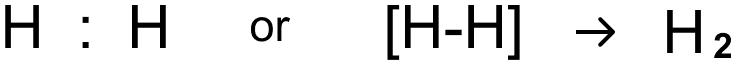(b) Formation of Chlorine (Cl2) — The valency of chlorine element is 1. It needs one electron to attain stable octet structure of nearest noble gas - Ar [2, 8, 8].

Each of the 'Cl' atoms contribute one electron so as to have one shared pair of electrons between them. Both atoms attain stable octet structure resulting in the formation of a single covalent bond [Cl-Cl] between them.

Electron Dot Structure:(c) Formation of Oxygen molecule (O2) — The valency of oxygen element is 2. It needs two electrons to attain stable octet structure of nearest noble gas - Ne [2,8].

Each of the two 'O' atoms contribute two electrons so as to have two shared pair of electrons between them. Both atoms attain stable octet structure resulting in the formation of a double covalent bond [O=O] between them.

Electron Dot Structure: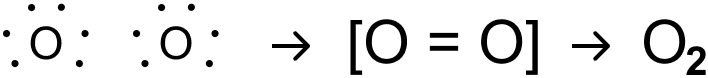(d) Formation of Nitrogen Molecule (N2) — The valency of nitrogen element is 3. Nitrogen needs three electrons to attain stable octet structure of nearest noble gas - Ne [2,8].

Each of the two N atoms contributes three electron so as to have three shared pair of electrons between them. Both atoms attain stable octet structure, resulting in the formation of a triple covalent bond [N≡N] between them.

Electron Dot Structure:(e) Formation of Water Molecule (H2O) — The valency of hydrogen element is 1 and that of oxygen is 2. Hydrogen needs one electrons to attain stable duplet structure of nearest noble gas - He  and oxygen needs two electrons to attain stable octet structure of nearest noble gas - Ne [2,8]

Each of the two hydrogen atoms shares an electron pair with the oxygen atom such that hydrogen acquires a duplet configuration and oxygen an octet configuration resulting in the formation of two single covalent bonds [H-O-H] in the molecule of water.

Electron Dot Structure:(f) Formation of Methane Molecule (CH4) — The valency of hydrogen element is 1 and that of carbon is 4. Hydrogen needs one electron to attain stable duplet structure of nearest noble gas - He  and carbon needs four electrons to attain stable octet structure of nearest noble gas - Ne [2,8]

One carbon atom shares four electron pairs one with each of the four atoms of hydrogen such that hydrogen acquires a duplet configuration and carbon an octet configuration resulting in the formation of four single covalent bonds.

Electron Dot Structure:(g) Formation of Carbon tetrachloride Molecule (CCl4) — The valency of carbon element is 4 and that of chlorine is 1. Chlorine needs one electron to attain stable octet structure of nearest noble gas - Ar [2,8,8] and Carbon needs four electrons to attain stable octet structure of nearest noble gas - Ne [2,8]

One carbon atom shares four electron pairs one with each of the four atoms of chlorine such that chlorine and carbon attain a stable octet configuration resulting in the formation of four single covalent bonds.

Electron Dot Structure:(h) Formation of Ammonia Molecule (NH3) — The valency of hydrogen element is 1 and that of nitrogen is 3. Hydrogen needs one electrons to attain stable duplet structure of nearest noble gas - He  and nitrogen needs three electrons to attain stable octet structure of nearest noble gas - Ne [2,8]

One nitrogen atom shares three electron pairs one with each of the three atoms of hydrogen such that hydrogen acquires a duplet configuration and carbon attain a stable octet configuration resulting in the formation of three single covalent bonds .

Electron Dot Structure: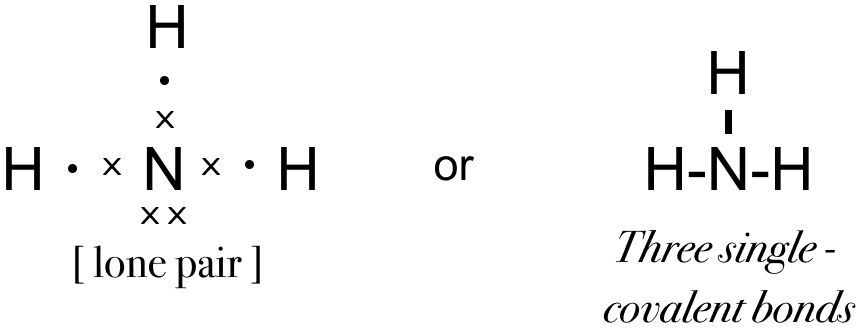(i) Formation of Carbon dioxide Molecule (CO2) — The valency of carbon element is 4 and that of oxygen is 2. Carbon needs four electrons to attain stable octet structure of nearest noble gas - Ne [2,8] and oxygen needs two electrons to attain stable octet structure of nearest noble gas - Ne [2,8]

One carbon atom shares two electron pairs one with each of the two atoms of oxygen such that oxygen and carbon attain a stable octet configuration resulting in the formation of two double covalent bonds.

Electron Dot Structure: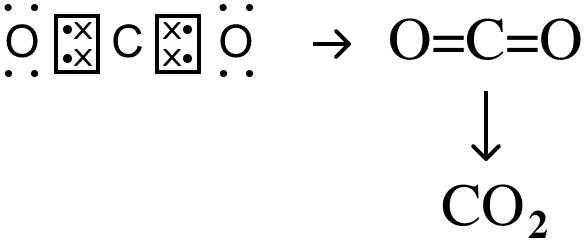#### Question 17.1

Give reasons for the following:

Molecules of hydrogen and chlorine have single covalent bonds between their atoms while oxygen has a double covalent and nitrogen a triple covalent bond respectively.

Depending on the number of electron pairs shared, covalent bond is single [-], double [=], or triple [≡] covalent.

Hydrogen — Each of the 'H' atoms contribute one electron so as to have one shared pair of electrons between them. Both atoms attain stable duplet structure resulting in the formation of a single covalent bond [H-H] between them.

Chlorine — Each of the two Cl atoms contributes one electron so as to have one shared pair of electrons between them. Both atoms attain stable octet structure, resulting in the formation of a single covalent bond [Cl–Cl] between them.

Oxygen — Each of the two O atoms contributes two electron so as to have two shared pair of electrons between them. Both atoms attain stable octet structure, resulting in the formation of a double covalent bond [O=O] between them.

Nitrogen — Each of the two N atoms contributes three electron so as to have three shared pair of electrons between them. Both atoms attain stable octet structure, resulting in the formation of a triple covalent bond [N≡N] between them.

#### Question 17.2

Give reasons for the following:

A molecule of methane has four single covalent bonds.

The valency of C is 4 and that of H is 1. Carbon needs four electrons to attain stable octet and hydrogen needs one electron to attain it's stable duplet state. Hence, one carbon atom shares four electron pairs - one with each of the four atoms of hydrogen. Hence, forms four single covalent bonds.

Electron Dot Structure :#### Question 17.3

Give reasons for the following:

Formation of ammonia involves one atom of nitrogen sharing three electron pairs one with each of the three atoms of hydrogen.

The valency of nitrogen is 3 and that of hydrogen is 1. N needs three electrons to attain a stable octet and H needs one electron to attain stable duplet. Hence, one atom of nitrogen shares three electron pairs one with each of the three atoms of hydrogen.

Electron Dot Structure:#### Question 18

Explain the terms :

(a) Lone pair of electrons

(b) Coordinate bond

Explain diagrammatically the lone pair effect of:

(a) The nitrogen atom of the ammonia molecule leading to the formation of ammonium ions [NH4]+

(b) The oxygen atom of the H2O molecule leading to formation of hydronium [H3O]+ and hydroxyl ions [OH]-

(a) Lone pair of electrons — They are a pair of electrons not shared with any other atom.

(b) Coordinate Bond — It is a type of covalency which involves one of the combining atoms contributing both of the shared electrons. i.e., a bond formed by a shared pair of electrons with both electrons coming from the same atom.

(a) Formation of ammonium ions [NH4]+In ammonia, the 'N' atom contains one lone pair of electrons after completing it's octet. This lone pair is accepted by the hydrogen ion of water leading to the formation of a coordinate covalent bond:

$-\overset{|}{\underset{|}{\text{N}}} \rarr \text{H}$

(b) Formation of hydronium (H3O)+ and hydroxyl ions [OH]-In water, the 'O' atom contains two lone pairs of electrons after completing it's octet. These lone pairs are accepted by the hydrogen ion leading to the formation of coordinate covalent bond:

$-\overset{|}{\text{O}}\rarr\text{H}$

#### Question 19

Give reasons for the following:

Electrovalent compounds are soluble in water, insoluble in organic solvents, good conductors of electricity in molten or aq. solution state, have high melting points and undergo electrolytic dissociation on passage of electric current, while covalent compounds are soluble in organic solvents, insoluble in water, non-conductors of electricity, have low melting points and undergo ionization on passage of electric current.

Electrovalent compounds

1. Solubility — Soluble in water, Insoluble in organic solvents.
Reason — Water [polar solvent] has a high dielectric constant i.e., capacity to weaken the force of attraction, thus resulting in free ions.
Organic solvents [non-polar] have low dielectric constants and do not cause dissolution.
2. Conduction of Electricity — Good conductors of electricity in molten or aqueous solution state and in solid state, they are non-conductors.
Reason — Strong electrostatic force keeps ions in fixed position in the solid state. The force is weakened in the molten state and disappears in aqueous solution state, hence free ions formed migrate to oppositely charged electrodes.
3. Melting Point — High melting point.
Reason — Strong electrostatic force of attraction between ions. Large amount of energy required to break the force of attraction.
4. Dissociation — Undergoes electrolytic dissociation on passage of electric current.
Reason — Process involves separation of ions already present in the ionic compound.
E.g. NaCl ⇌ Na1+ + Cl1- [in molten state]

Covalent compounds

1. Solubility — Soluble in organic solvents [non-polar] but insoluble in water [polar].
Reason — Organic solvents [non-polar] e.g., the benzene, alcohol, dissolves non-polar covalent compounds [like dissolves like]. Water [polar solvent] cannot dissolve non-polar compounds, but dissolves polar.
2. Conduction of Electricity — Non-conductors of electricity.
Reason — Non-polar compounds e.g., CCl4 contain molecules and not free ions. Hence, are non conductors. Polar covalent compounds e.g., HCl, NH3 show charge seperation and dissociate in H2O.
3. Melting Point —Low melting points.
Reason — Weak Vander Waals force of attraction between molecules. Thus, less amount of energy is required to break these forces of attraction.
4. Ionization — Undergoes ionization in solution state on passage of electric current. Process involves formation of ions from molecules which are not in ionic state.
E.g. HCl ⟶ H1+ + Cl1- [in solution state]

## Unit Test Paper 2 — Chemical Bonding

#### Question 1.1

Give reasons for the following:

NH3 gas a covalent compound does not conduct electricity but it's aq. soln. NH4OH is a weak electrolyte.

NH3 gas is a covalent compound hence, there are no ions present in it and it does not conduct electricity.
When NH3 gas is dissolved in water it forms an aqueous solution of ammonium hydroxide.
The ammonium hydroxide formed is a weak electrolyte because it can ionise to a small extent to form ammonium ions and hydroxide ions. These ions are mobile and hence, conduct electricity.

#### Question 1.2

Give reasons for the following:

MgCl2 is soluble in water but insoluble in acetone, while methane is insoluble in water, but soluble in acetone.

MgCl2 is a electrovalent compound, so it is soluble in water (as water is a polar solvent and has a high dielectric constant) and insoluble in organic solvents like acetone (as organic solvents are non-polar and have low dielectric constant and do not cause dissolution).
Methane is a covalent compound and is insoluble in polar solvents like water and dissolve in organic (non-polar) solvents like acetone.

#### Question 1.3

Give reasons for the following:

Iron displaces copper from a solution of a copper salt. The reaction is deemed as a redox reaction.

When iron displaces copper from a solution of a copper salt, then the reaction is as follows:

Fe + CuSO4 ⟶ FeSO4 + Cu

Ionic equation for the reaction can be written as:

Fe + Cu2+ ⟶ Fe2+ + Cu

Iron loses two electrons and is oxidized to Fe2+.

Fe ⟶ Fe2+ [Oxidation] [-2e-]

Cu2+ gains two electrons and is reduced to copper.

Cu2+ ⟶ Cu [Reduction] [+2e-]

Thus, the given reaction is a redox reaction.

#### Question 1.4

Give reasons for the following:

A non-metallic atom (at. no. 9) forms a molecule of the same, containing a single covalent bond.

Electronic configuration of the non-metallic element with atomic number 9 is = (2,7)

In order to complete it's octet and attain a stable state it requires one electron hence, one electron from the valence shell of two such atoms are mutually shared such that each atom acquires a stable electronic configuration resulting in the formation of single covalent bond between them.

The diagrammatic representation is shown below: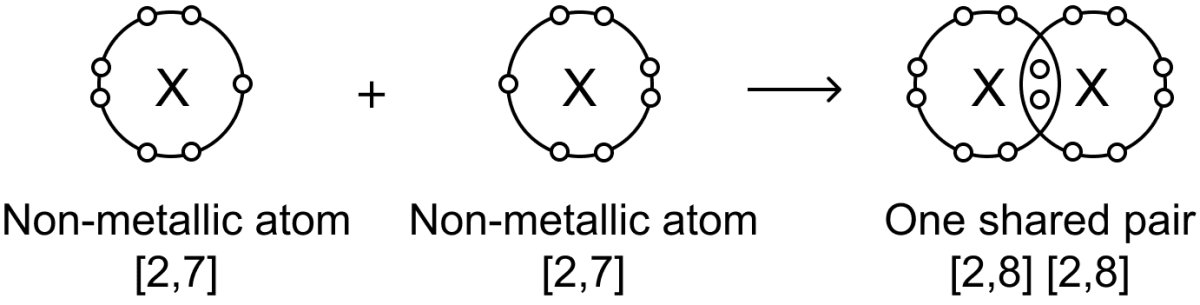#### Question 1.5

Give reasons for the following:

In the formation of MgO the magnesium atom (at. no. 12) loses two electrons from it's valence shell.

Electronic configuration of Mg (Z = 12) = 2, 8, 2

Electronic configuration of O (Z = 8) = 2, 6

Mg (metal) has a tendency to lose two valence electrons and attain a stable state (Ne = 2,8). On the other hand, O (non-metal) has a tendency to gain two electron and attain a stable state (Ne = 2,8). Hence, when the two atoms fuse together to form MgO, then Mg loses two electrons and O gains two electrons and they form a electrovalent bond.

#### Question 2

With reference to a molecule of water, fill in the blanks with the correct word. [at. no. of H = 1, O = 8]

Water is a ............... [non-polar/polar] covalent molecule in which the atom of ............... [hydrogen/oxygen] attracts electrons more strongly towards itself. The water molecule shows the presence of ............... [double / one single / two single] covalent bond/s and ............... [one/two] lone pair of electrons present in the ............... [hydrogen/oxygen] atom.

Water is a polar covalent molecule in which the atom of oxygen attracts electrons more strongly towards itself. The water molecule shows the presence of two single covalent bond/s and two lone pair of electrons present in the oxygen atom.

#### Question 3

Complete the table given below:

ElementElementType of bond likely in molecule ofFormula of compound
A: At. no. = 20B: At no. = 8A and B
A: At no. = 20C: At no. = 17A and C
D: At. no. = 9D: At. no. = 9D and D
E: At. no. = 12F: At. no. = 16E and F
G: At. no. = 1F: At. no. = 16G and F

ElementElementType of bond likely in molecule ofFormula of compound
A: At. no. = 20B: At no. = 8A and B → Electrovalent bondAB (CaO)
A: At no. = 20C: At no. = 17A and C → Electrovalent bondAB2 (CaCl2)
D: At. no. = 9D: At. no. = 9D and D → Covalent bondD2 (F2)
E: At. no. = 12F: At. no. = 16E and F → Electrovalent bondEF (MgS)
G: At. no. = 1F: At. no. = 16G and F → Covalent bondG2F (H2S)

#### Question 4

Fill in the blanks with the correct word from the brackets:

1. The bond between two elements in group 17[VIIA] of the periodic table is likely to be ............... [ionic/covalent].
2. In the reaction of Cl2 + 2KI ⟶ 2KCl + I2 the conversion of 2I to I2 is deemed as ............... [oxidation/reduction].
3. The covalent molecule containing three single covalent bonds is ............... [water/methane/ammonia].
4. The molecule of water combines with a ............... [hydrogen atom/proton/hydrogen molecule] to form a hydronium ion.
5. For formation of an electrovalent bond between elements 'X' and 'Y' which are a metal and non-metal respectively, X should have a ............... [high/low] ionization potential and 'Y' a ............... [high/low] electron affinity.

1. The bond between two elements in group 17[VIIA] of the periodic table is likely to be covalent.
2. In the reaction of Cl2 + 2KI ⟶ 2KCl + I2 the conversion of 2I to I2 is deemed as oxidation.
3. The covalent molecule containing three single covalent bonds is ammonia.
4. The molecule of water combines with a proton to form a hydronium ion.
5. For formation of an electrovalent bond between elements 'X' and 'Y' which are a metal and non-metal respectively, X should have a low ionization potential and 'Y' a high electron affinity.

#### Question 5

Electronic configuration of the following elements are given:

1. Sodium — Na = 2, 8, 1
2. Hydrogen — H = 1
3. Carbon — C = 2, 4
4. Chlorine — Cl = 2, 8, 7
5. Lithium — Li = 2,1

State which of the compounds given below:

(a) have high/low boiling points,
(b) are soluble/insoluble in organic solvents.

A : Hydrogen chloride
B : Sodium chloride
C : Sodium hydride
D : Lithium chloride
E : Carbon tetrachloride

(a) Compounds having high/low boiling points are —

• A : Hydrogen chloride → low boiling point
• B : Sodium chloride → high boiling point
• C : Sodium hydride → high boiling point
• D : Lithium chloride → high boiling point
• E : Carbon tetrachloride → low boiling point

(b) Compounds which are soluble/insoluble in organic solvents.

• A : Hydrogen chloride → insoluble in organic solvents.
• B : Sodium chloride → insoluble in organic solvents.
• C : Sodium hydride → insoluble in organic solvents.
• D : Lithium chloride → insoluble in organic solvents.
• E : Carbon tetrachloride → soluble in organic solvents.

#### Question 6

A compound has a formula = 'H2Y'. Y denotes a non-­metal. State the following:

1. The electronic configuration of Y.
2. The valency of Y.
3. The bonding present in 'H2Y'
4. The bonding present in the compound formed between potassium ($^{39}_{19}\text{K}$) and 'Y'
5. The formula of the compound formed between calcium ($^{40}_{20}\text{Ca}$) and Y.

4. Ionic bond is present between potassium ($^{39}_{19}\text{K}$) and 'Y' because K is a metal and Y is a non-metal.
5. CaY is the formula for the compound formed between calcium ($^{40}_{20}\text{Ca}$) and Y by transfer of two valence electrons. (As the electronic configuration of Y matches that of Oxygen so the actual formula will be CaO)